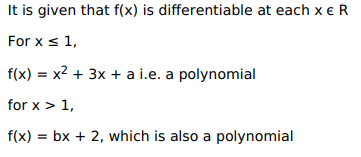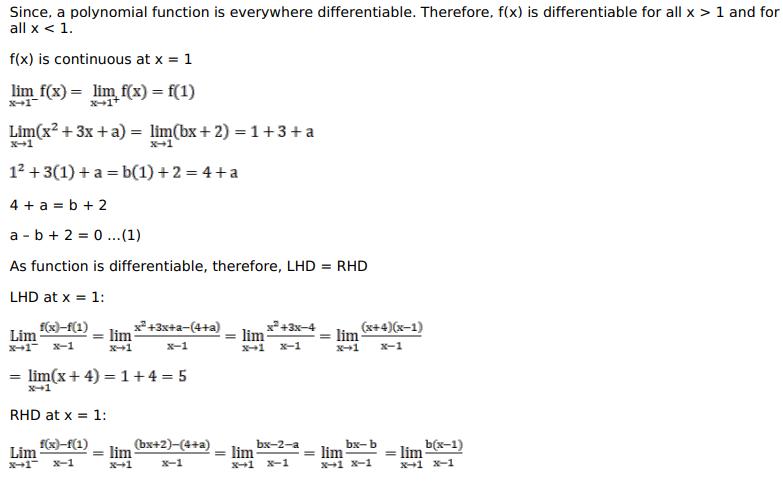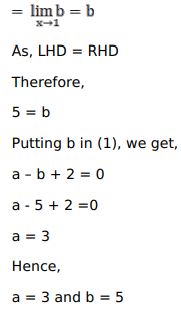# Find the values of a and b so that the functionQuestion:

Find the values of a and b so that the function

$f(x)=\left\{\begin{array}{c}\left(x^{2}+3 x+a\right), \text { when } x \leq 1 ; \\ (b x+2), \text { when } x>1\end{array}\right.$ is differentiable at each $x \in R$

Solution: